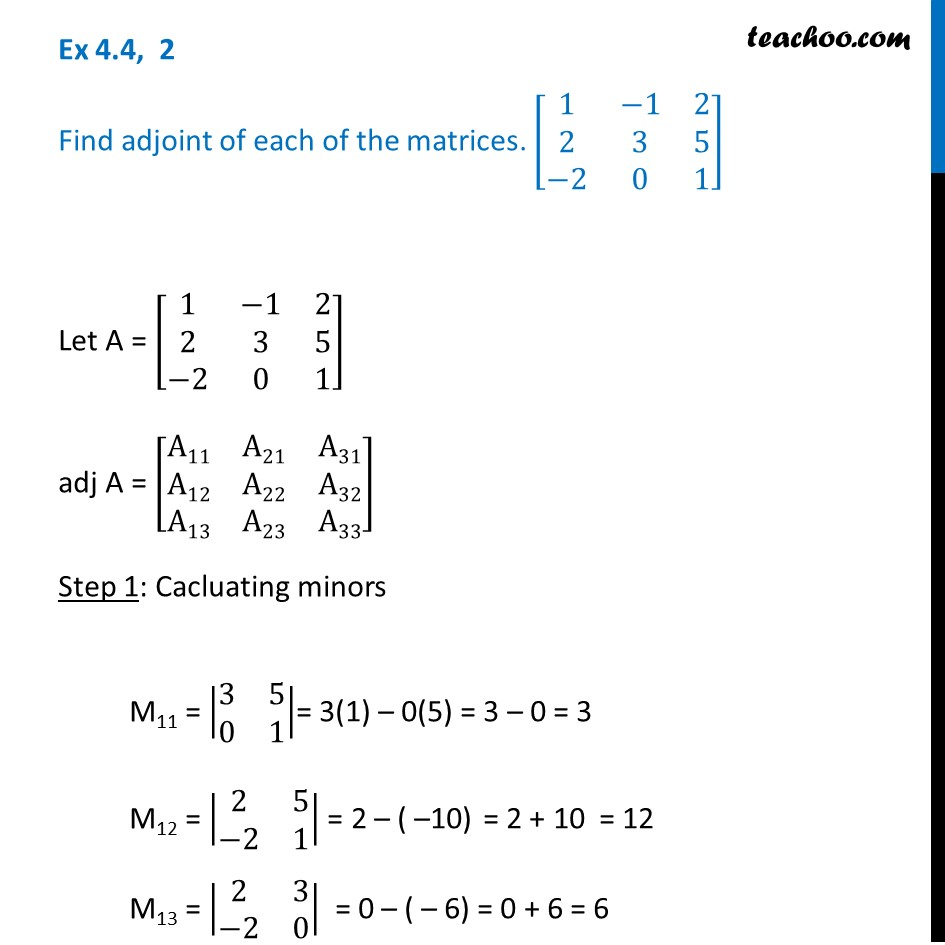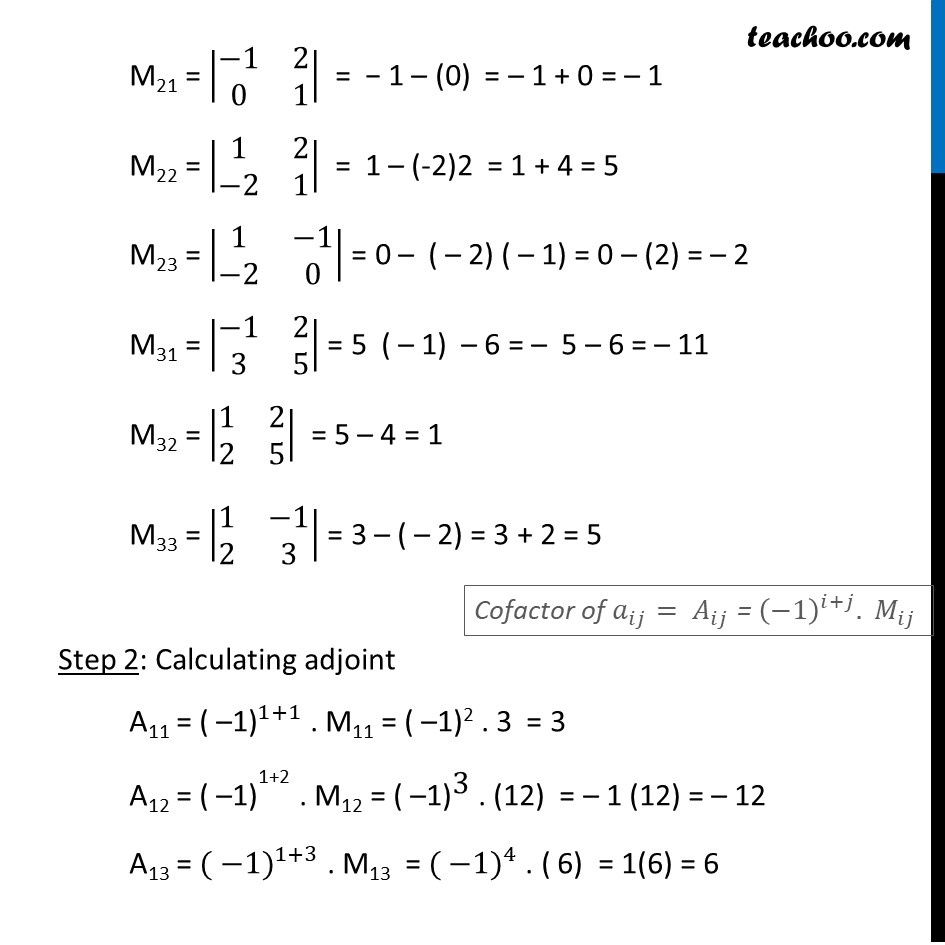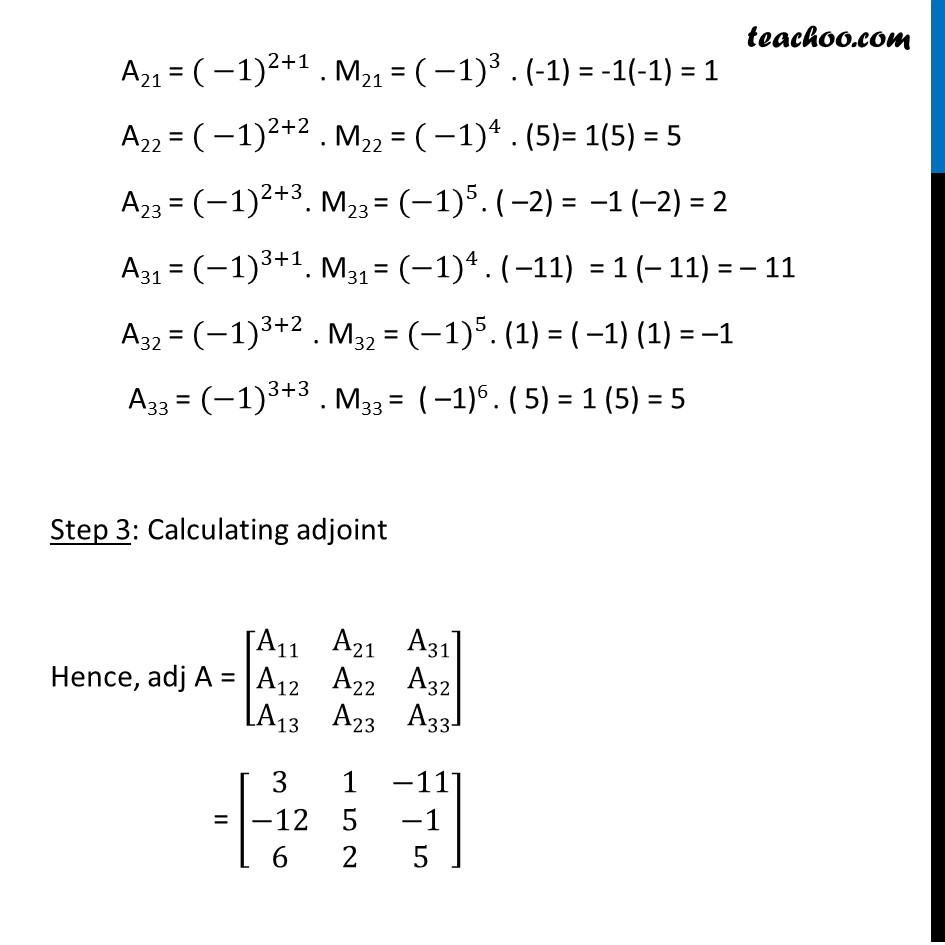Ex 4.4

Chapter 4 Class 12 Determinants
Serial order wiseLearn in your speed, with individual attention - Teachoo Maths 1-on-1 Class

### Transcript

Ex 4.4, 2 Find adjoint of each of the matrices. [■8(1&−1&2@2&3&5@−2&0&1)] Let A = [■8(1&−1&2@2&3&5@−2&0&1)] adj A = [■8(A11&A21&A31@A12&A22&A32@A13&A23&A33)] Step 1: Cacluating minors M11 = |■8(3&5@0&1)|= 3(1) – 0(5) = 3 – 0 = 3 M12 = |■8(2&5@−2&1)| = 2 – ( –10) = 2 + 10 = 12 M13 = |■8(2&3@−2&0)| = 0 – ( – 6) = 0 + 6 = 6 M21 = |■8(−1&2@0&1)| = − 1 – (0) = – 1 + 0 = – 1 M22 = |■8(1&2@−2&1)| = 1 – (-2)2 = 1 + 4 = 5 M23 = |■8(1&−1@−2&0)| = 0 – ( – 2) ( – 1) = 0 – (2) = – 2 M31 = |■8(−1&2@3&5)| = 5 ( – 1) – 6 = – 5 – 6 = – 11 M32 = |■8(1&2@2&5)| = 5 – 4 = 1 M33 = |■8(1&−1@2&3)| = 3 – ( – 2) = 3 + 2 = 5 Step 2: Calculating adjoint A11 = 〖"( –1)" 〗^(1+1) . M11 = ( –1)2 . 3 = 3 A12 = 〖"( –1)" 〗^"1+2" . M12 = 〖"( –1)" 〗^"3" . (12) = – 1 (12) = – 12 A13 = 〖( −1)〗^(1+3) . M13 = 〖( −1)〗^4 . ( 6) = 1(6) = 6 A21 = 〖( −1)〗^(2+1) . M21 = 〖( −1)〗^3 . (-1) = -1(-1) = 1 A22 = 〖( −1)〗^(2+2) . M22 = 〖( −1)〗^4 . (5)= 1(5) = 5 A23 = 〖(−1)〗^(2+3). M23 = 〖(−1)〗^5. ( –2) = –1 (–2) = 2 A31 = 〖(−1)〗^(3+1). M31 = 〖(−1)〗^4 . ( –11) = 1 (– 11) = – 11 A32 = 〖(−1)〗^(3+2) . M32 = 〖(−1)〗^5. (1) = ( –1) (1) = –1 A33 = 〖(−1)〗^(3+3) . M33 = ( –1)6 . ( 5) = 1 (5) = 5 Step 3: Calculating adjoint Hence, adj A = [■8(A11&A21&A31@A12&A22&A32@A13&A23&A33)] = [■8(3&1&−11@−12&5&−1@6&2&5)]